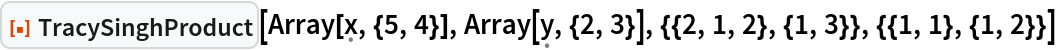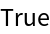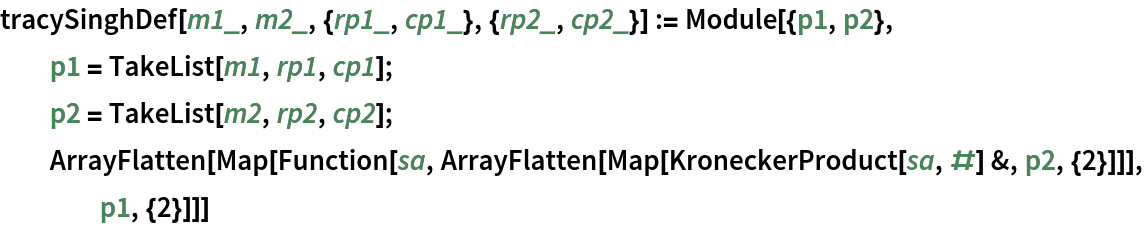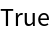Function Repository Resource:

# TracySinghProduct

Calculate the Tracy–Singh product of two matrices

Contributed by: Jan Mangaldan
 ResourceFunction["TracySinghProduct"][m1,m2,{r1,c1},{r2,c2}] gives the Tracy-Singh product of m1 and m2, where the ri and ci are corresponding integer partitions of the dimensions of the mi.

## Details

If m1 has dimensions p1×q1, then r1 and c1 must both be vectors with positive integer components that respectively sum top1 and q1, and similarly for m2.
ResourceFunction["TracySinghProduct"] can be used on SparseArray objects, returning a SparseArray object when possible.

## Examples

### Basic Examples (1)

The Tracy-Singh product of two partitioned matrices:

 In:=Out=### Scope (2)

The Tracy-Singh product of two rectangular matrices with symbolic entries:

 In:=Out=The Tracy-Singh product of two sparse matrices:

 In:=Out=### Properties and Relations (2)

The Kronecker product is a special case of the Tracy-Singh product:In:=Out=In:=Out=The Tracy-Singh product is effectively the pairwise Kronecker product for each pair of partitions in the two matrices:In:=Out=## Version History

• 1.0.0 – 19 August 2022